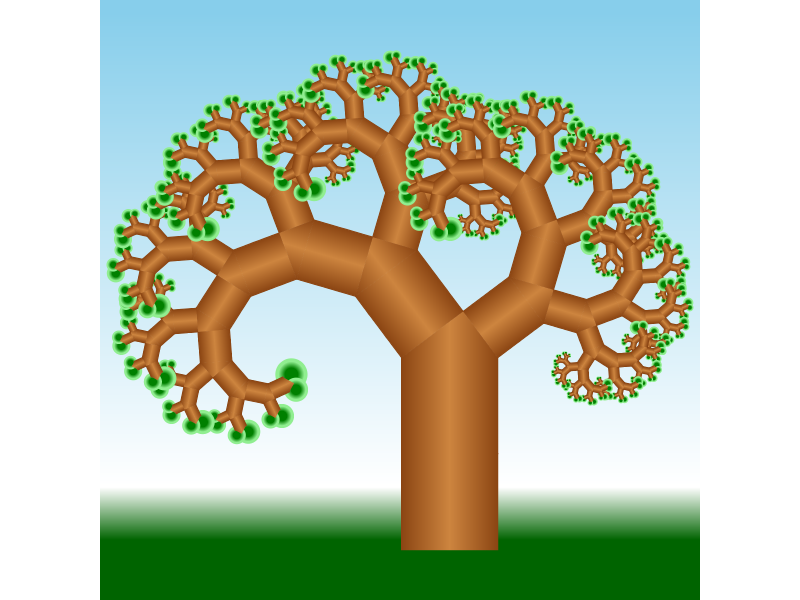A fractal made of squares and right-angled triangles that looks like a tree.Author: Chris Mears
> import Diagrams.Backend.SVG.CmdLine

This diagram was inspired by the one at http://projecteuler.net/problem=395, which explains the algorithm for constructing the tree.

> {-# LANGUAGE NoMonomorphismRestriction #-}
> import Diagrams.Prelude
> import Diagrams.TwoD
> import Data.Colour (blend)

An order $$n$$ tree has a square and a right-angled triangle on top, and an order $$n-1$$ tree on each short side of the triangle. As the recursion deepens, the limbs get smaller and more transparent.

For aesthetics, let the leaves have circles instead of squares.

> stops = mkStops [(saddlebrown, 0, 1), (peru, 0.5, 1), (saddlebrown, 1, 1)]
> b = mkLinearGradient stops ((-0.5) ^& 0) (0.5 ^& 0) GradPad
> stops' = mkStops [(green, 0, 1), (lightgreen, 1, 1)]
> g = mkRadialGradient stops' (0.0 ^& 0) 0.5 (0.0 ^& 0.0) 1 GradPad
> sky = mkLinearGradient (mkStops [(darkgreen,0,1), (white,0.1,1), (skyblue,1,1)])
>                        (0 ^& (-2.5)) (0 ^& 3) GradPad
> tree 1 = circle 1.25 # fillTexture g
>                     # translate (r2 (0, 1/2)) # lwG 0
> tree n =
>   square 1          # translate (r2 (0, 1/2)) # fillTexture b
>                     # lineTexture b # lw thin
>   atop triangle   # translate (r2 (0,1))    # fillTexture b # lwG 0
>   atop tree (n-1) # rotate (-asin 0.8 @@ rad)
>                     # scale 0.6 # translate (r2 ( 0.32,1.24)) # fade
>   atop tree (n-1) # rotate ( asin 0.6 @@ rad)
>                     # scale 0.8 # translate (r2 (-0.18,1.24)) # fade
>   where
>     triangle = translate (r2 (-0.5,0)) . strokeLoop . closeLine
>                  . fromVertices . map p2 \$ [(0,0), (1,0), (0.8*0.8,0.8*0.6)]
> colourise c = fc c . lc (blend 0.5 black c)
> example = (tree 10 === square 1 # fillTexture b
>                     <> (square 6.25 # fillTexture sky # lw none )
> main = mainWith (example :: Diagram B)